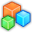# Microsoft Small BasicProgram Listing: JPW517
'Finds the start and end dates of British Summer Time.

wlft = 560
wtop = 160
'
clft = 32
ctop = 8

TextWindow.CursorLeft = clft
TextWindow.CursorTop = ctop
TextWindow.Write("Dates of BST for the year ")

'Find the day BST starts:-
J = 1721150.5
StartFinish = "Starts on "
Month = " March at 1 a.m. GMT"
a = 3
b = 5
BST()

'Find the day BST finishes:-
J = 1721364.5
StartFinish = "Ends on "
Month = " October at 2 a.m. BST."
a = 8
b = 10
BST()

sub BST
ym = yr + 1
S = Math.Floor( 7 * ym / 4) + Math.Floor(3 * ((ym + 99) / 100) / 4)
JD31 = -S + 367 * yr + J
DWk = Math.Remainder(Math.Floor(JD31 + .5),7) 'The day of the week of the 31st of the month.
IF DWk = 6 THEN
DayDiff = 0
ELSE
DayDiff = -(1 + DWk)
ENDIF 'giving the difference in days between the last Sunday & the 31st.
LimitDay = DayDiff + 31
LimitJD = .04167 + DayDiff + JD31
TextWindow.CursorLeft = clft
TextWindow.CursorTop = ctop + a
TextWindow.WriteLine(StartFinish + LimitDay + Month)
TextWindow.CursorLeft = clft
TextWindow.CursorTop = ctop + b
TextWindow.WriteLine("JD = " + LimitJD)
TextWindow.CursorLeft = clft
TextWindow.CursorTop = ctop + 13
EndSub